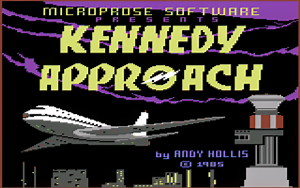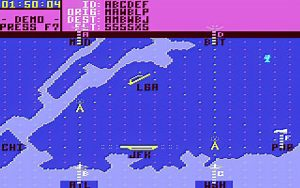# ELITE – Object ID Sheet

ELITE by FIREBIRD just seems to ADVANCE for 1985.
There was a few different versions with different copy protection.
Here in the USA one verions was GMA with extra tracks 36-40
Another version used Lenslock and a manual Symbol look up.

# Die Hard 2 – Code Sheet

Die Hard 2 used a code sheet for it’s copy protection.
The game will ask you for the arrival time of a flight and here they are:

ETAs for Die Hard 2 – Die Harder:

1. PC029 = 0509
2. BA123 = 1200
3. DA456 = 1330
4. BC789 = 1420
5. SA012 = 0955
6. TH256 = 0417
7. PC048 = 0258
8. MT369 = 0035
9. MC252 = 1939
10. LQ159 = 1830
11. ME357 = 1945
12. PV029 = 2036
13. AO146 = 1655
14. LZ358 = 2345
15. JW012 = 1409
16. JK356 = 0256
17. PA569 = 0652
18. AA143 = 1708
19. IS692 = 2120
20. JM713 = 1555
21. MW258 = 1618
22. PX016 = 0856
23. OT657 = 2234
24. AM359 = 0327
25. ST845 = 1102
26. VE379 = 0913
27. DA014 = 2345
28. IC391 = 1831
29. LS089 = 0600
30. JE473 = 2126
31. HJ193 = 0908
32. UI384 = 0548

# Kennedy Approach Code SheetKennedy Approach is such a fun game. Which I had spent hours and hours playing.Before you start the game you needed to enter a password.

It’s a shirt list, but here it is:

Kennedy Approach – Commodore 64
This game uses a code sheet:

1 – DME
2 – VOR
3 – ATC
4 – FAR
5 – IAP
6 – GCA
7 – FSS
8 – HAT
9 – VFR
10 – STAR
11 – SID
12 – JFR
13 – TDZ
14 – PAR
15 – NOB
16 – ELT